﻿ 220 kV双回塔检修避雷器带电作业电场仿真研究

# 220 kV双回塔检修避雷器带电作业电场仿真研究Electric Field Simulation Study on Live Working of Overhauling Arrester on 220 kV Double Circuit Tower

Abstract: In order to improve the reliable operation level of overhead transmission lines, it is necessary to carry out regular overhaul of arresters in the southwest of China by installing arresters on 220 kV transmission lines. In order to ensure the safety of operators in live working, the relevant model is established in SolidWorks software, and the finite element method is used to simulate the live working of 220 kV Double Circuit Tower overhaul arrester, i.e. equipotential operation and three ways of entering equipotential operation. The distribution of electric field on the human body surface of live workers is obtained and analyzed, and then three ways of entering equipotential operation are compared, which can provide reference for the personal safety of live workers.

1. 引言

2. 带电作业电场计算方法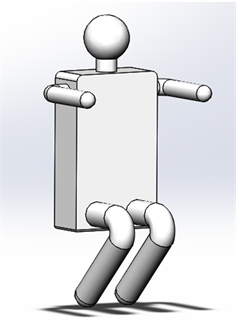Figure 1. Human Model of Operators in Live Working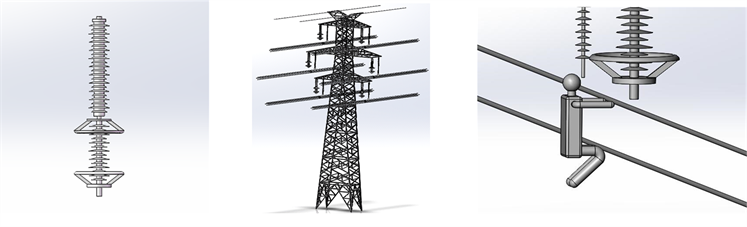(a) 支撑间隙避雷器模型(b) 220 kV带支撑间隙避雷器双回路塔模型 (c) 等电位作业人体坐姿模型

Figure 2. SolidWorks Models

$\nabla ×E=0$ (1)

$\nabla \cdot D=\rho$ (2)

$D=\epsilon E$ (3)

$E=-\nabla \phi$ (4)

$\nabla \cdot \left(\epsilon E\right)=\rho$ (5)

$\nabla \cdot \left(-\epsilon \nabla \phi \right)=\rho$ (6)

$\epsilon {\nabla }^{2}\phi +\nabla \phi \cdot \nabla \epsilon =-\rho$ (7)

${\nabla }^{2}\phi =-\rho /\epsilon$ (8)

$\frac{{\partial }^{2}\phi }{{\partial }^{2}x}+\frac{{\partial }^{2}\phi }{{\partial }^{2}y}+\frac{{\partial }^{2}\phi }{{\partial }^{2}z}=-\frac{\rho }{\epsilon }$ (9)

$\frac{{\partial }^{2}\phi }{{\partial }^{2}x}+\frac{{\partial }^{2}\phi }{{\partial }^{2}y}+\frac{{\partial }^{2}\phi }{{\partial }^{2}z}=0$ (10)

3. 仿真计算

3.1. 等电位带电作业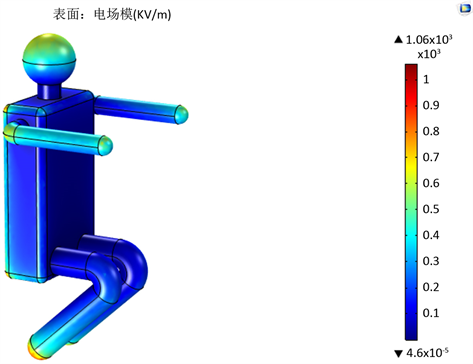Figure 3. Electric field distribution map of human body surface in equipotential operationTable 1. Surface Electric Field Strength of Operators at Equipotential Working (kV/m)

$SE=20\mathrm{lg}\left(\frac{{U}_{ref}}{U}\right)$ (11)

GB/T 6568-2008《带电作业用屏蔽服装》规定，整套屏蔽服在规定的使用电压等级下，衣服内的体表场强不得大于15 kV/m，人体外露部位的体表局部场强不得大于240 kV/m。考虑到人体表面出现的最大场强为1100 kV/m，穿戴60 dB的屏蔽服时，服内场强为1.1 kV/m，能满足带电作业的要求。作业人员面部不可以裸露，需穿戴20 dB的屏蔽面罩。

3.2. 进入等电位带电作业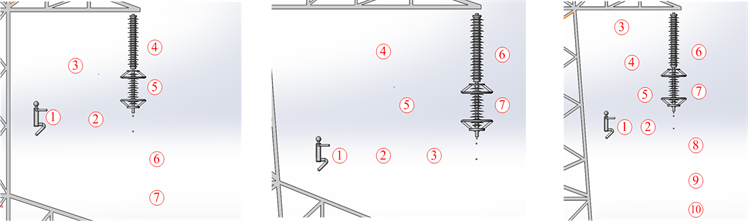(a)上相 (b)中相 (c)下相

Figure 4. Typical Position Diagram for Entering Equipotential Operation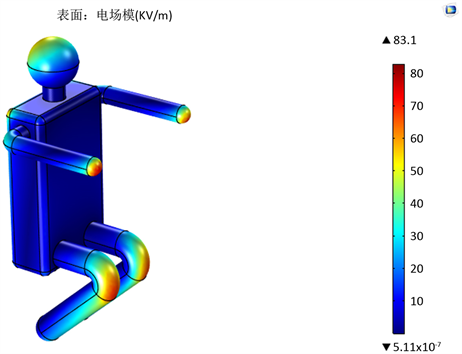Figure 5. Electric Field Distribution on Human Body Surface at Entering Equipotential OperationTable 2. Surface Electric field strength of Operators entering equipotential operation (kV/m)

4. 总结

 王海龙. 准确把握形势, 紧密结合改革 统筹做好2019年全国电力需求侧管理工作[J]. 电力需求侧管理, 2019, 21(1): 1-3.

 刘振亚. 中国电力与新能源[M]. 北京: 中国电力出版社, 2012.

 胡毅, 刘凯, 彭勇, 等. 带电作业关键技术研究进展与趋势[J]. 高电压技术, 2014, 40(7): 1921-1931.

 胡毅. 输配电线路带电作业技术的研究与发展[J]. 高电压技术, 2006, 32(11): 1-10.

 李春华. 输配电线路带电作业技术的应用研究[J]. 技术与市场, 2018, 25(12): 105-106.

 虢韬, 梁文勇, 杨恒, 等. 基于全息数据的贵州电网雷击故障诊断研究[J]. 陕西电力, 2015, 43(4): 51-55.

 季坤, 严波, 操松元, 等. 220 kV同塔双回输电线路避雷器防护配置研究[J]. 电瓷避雷器, 2018, 284(4): 128-137.

 苏杰, 曹炯, 姜文东, 等. 220 kV/110 kV同塔四回输电线路双回同时闪络耐雷性能及防治[J]. 电瓷避雷器, 2013(5): 90-96.

 李振, 余占清, 何金良, 等. 线路避雷器改善同塔多回线路防雷性能的分析[J]. 高电压技术, 2011, 37(12): 3120-3128.

 曾赟, 漆铭钧, 李婷, 等. 基于不停电检测的避雷器状态检修优化研究[J]. 湖南电力, 2016, 36(3): 19-22.

 陈坤汉, 李顺尧. 一起220 kV线路避雷器泄漏电流超标的原因分析[J]. 高压电器, 2012, 48(5): 111-114.

 刘凯, 吴田, 刘庭, 等. ±800 kV特高压直流输电线路的电位转移电流特性[J]. 高电压技术, 2013, 39(10): 568-576.

 方雅琪, 彭勇, 苏梓铭, 等. 特高压紧凑型线路带线作业人体表电场仿真计算[J]. 中国电力, 2015, 48(10): 77-83.

 胡毅, 胡建勋, 刘凯, 等. 特高压交直流线路带电作业人员的体表场强[J]. 高电压技术, 2010, 36(1): 13-18.

Top